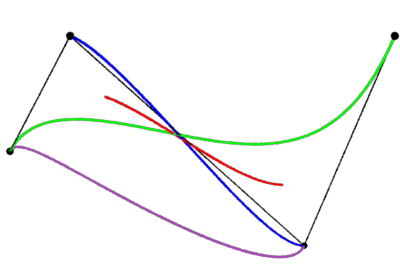### RenderManCurve Basics

##### References

"Nurb Curves: A Guide for the Uninitiated"
`http://devworld.apple.com/dev/techsupport/develop/issue25/schneider.html`

#### Introduction

This tutorial covers the basic issues of dealing with the RenderMan's curve primitive. Figure 1 shows four colored `Curves`, that have been rendered using different "curve types" but sharing identical control vertices (cv's).

```    b-spline
bezier
catmull-rom
hermite```

The cv's are colored white and gray connecting lines show the sequence of cv's.Figure 1

There is a clear difference between each of the curves. In particular, notice the green bezier curve is the only one to begin and end at the first and last cv. The RIB statements used to render the bezier curve are,

```Basis "bezier" 3 "bezier" 3
Curves "cubic"  "nonperiodic"
"P" [-0.75 0 0.5  -0.45 0 1  0.5 0 0  0.75 0 1]
"constantwidth" [0.005]
"Cs" [0 1 0   0 1 0]```

The rib file used to render this image can be viewed here. Curves generated by Maya and mtor/Rfm the curve type is "b-spline" ie.

`    Basis "b-spline" 1 "b-spline" 1`

Refer to rendering Maya curves with prman.

#### Assumptions

Although a RenderMan curve might look as if it can be defined by an arbitary number of cv's - in the example shown above the "`P`" list contains four lots of xyz's. Infact, the number of cv's must conform to formula determined by the type of curve being rendered. The simpliest curve type to use is a "b-spline" because it can be defined by any number of cv's - greater than 4. Unfortunately, in general a "b-spline" curve does not begin and end at the first and last cv.

A "bezier" curve does have the desirable property of starting and finishing at the first and last cv. However, the number of cv's, less 1, must be exactly divisible by 3. For instance, we might use 4, 7, 10 or 13 cv's for a "bezier" curve. The only time when the restriction on the number of cv's can be ignored is when a "periodic" curve is produced, in which case, the end of the curve wraps around to coincide with the beginning of the curve.

A single RenderMan `Curves` statement can define multiple separate curves. A function in the 'C' programming language is presented next that, for simplicity, generates a single curve. The function can be called multiple times in order to generate hundreds if not thousands of curves.

#### 'C' Code

The code for a function called filament() is shown below. It produces a "nonperiodic" curve ie. the end of the curve does not wrap around to the beginning of the curve. The number of cv's passed to this function must conform to the bezier constraint that,

`    (numCV - 1) / 3`

divides without a remainder. The function returns 1 if the numCV it has been given satisfies this constraint, otherwise it returns zero to indicate an error.

Listing 1

 ```int filament(int numCV, float cv[], float rgb[], float thickness) { int segments; /* check the number of cv's */ if(numCV - 1) % 3 != 0) return 0; /* "open" the Curves statement */ printf("Curves \"cubic\" [%d] \"periodic\" \"P\" [", numCV); /* add the list of cv's */ for(n = 0; n < numCV; n++) printf("%f %f %f ", cv[n], cv[n], cv[n]); /* for simplicity use uniform thickness */ printf("] \"constantwidth\" [%f]\n", thickness); /* "open" the surface color parameter */ printf("\"Cs\" ["); /* add the list of colors */ segments = (numCV - 1)/3; for(n = 0; n < segments + 1; n++) printf("%f %f %f ", rgb[n], rgb[n], rgb[n]); /* "close" the Curves statement */ printf("] \n"); return 1; } ```